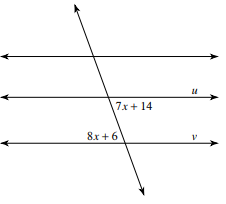# Equations involving angle relationships - Part 1

## Determining variable values that make lines parallel

The relationships between angles within parallel lines and a transversal are well known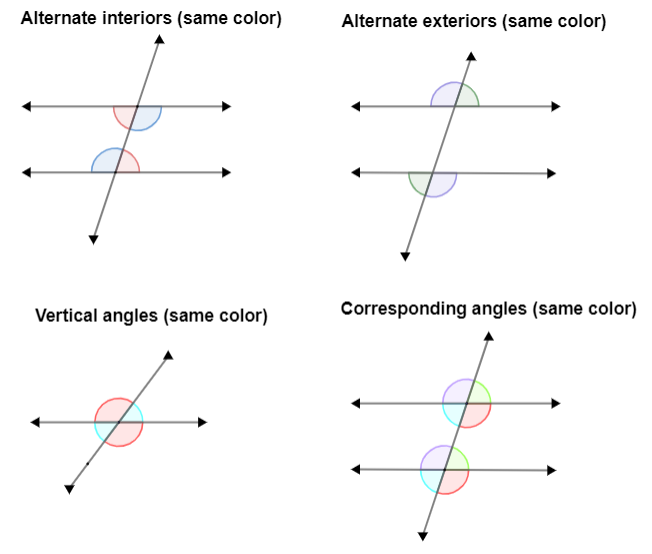To determine variable values that make lines parallel, you should consider what pair of congruent angles do you have, and solve an equation that shows that their measures are equivalent. Example: If the angles whose measures are and , are congruent, then: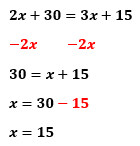Then, the measure of the angle is or

## Find the value of x that makes lines u and v parallel. Show your work step by step.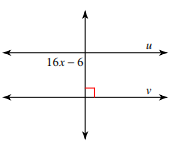## Find the value of x that makes lines u and v parallel. Show your work step by step.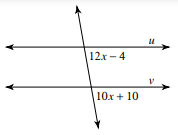## Find the value of x that makes lines u and v parallel. Show your work step by step.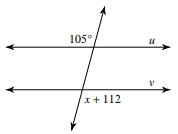## Find the value of x that makes lines u and v parallel. Show your work step by step.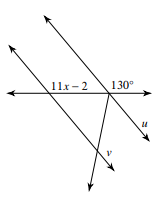## What value of x will make lines u and v intersect? Explain